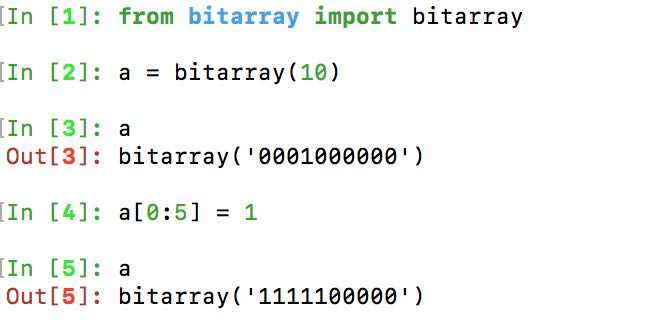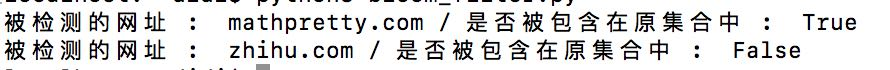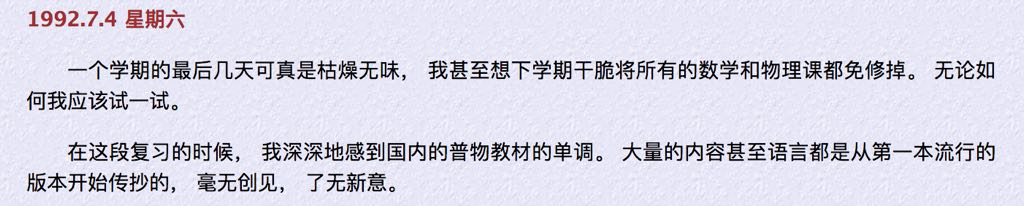# Python实现布隆过滤器

• A+

## 代码及运行结果1. import mmh3
2. from bitarray import bitarray
3. # Implement a simple bloom filter with murmurhash algorithm.
4. # Bloom filter is used to check wether an element exists in a collection, and it has a good performance in big data situation.
5. # It may has positive rate depend on hash functions and elements count.
6. class BloomFilter(object):
7.     def __init__(self,BIT_SIZE):
8.         # 初始化布隆过滤器,生成一下全0的 bitarray
9.         bit_array = bitarray(BIT_SIZE)
10.         bit_array.setall(0)
11.         self.bit_array = bit_array
13.         # 添加一个url,同时获取这个url的对应的bitarray的位置
14.         point_list = self.get_postions(url)
15.         for b in point_list:
16.             self.bit_array[b] = 1
17.     def contains(self, url):
18.         # 验证这个url是否存在在集合中
19.         point_list = self.get_postions(url)
20.         result = True
21.         for b in point_list:
22.             result = result and self.bit_array[b]
23.         return result
24.     def get_postions(self, url):
25.         # 一个url获取七个位置,之后会把这七个位置变为1
26.         point1 = mmh3.hash(url, 41) % BIT_SIZE
27.         point2 = mmh3.hash(url, 42) % BIT_SIZE
28.         point3 = mmh3.hash(url, 43) % BIT_SIZE
29.         point4 = mmh3.hash(url, 44) % BIT_SIZE
30.         point5 = mmh3.hash(url, 45) % BIT_SIZE
31.         point6 = mmh3.hash(url, 46) % BIT_SIZE
32.         point7 = mmh3.hash(url, 47) % BIT_SIZE
33.         return [point1, point2, point3, point4, point5, point6, point7]
34. if __name__ == '__main__':
35.     BIT_SIZE = 5000000
36.     # 类的实例化
37.     bloom_filter = BloomFilter(BIT_SIZE)
38.     urls = ['www.baidu.com','mathpretty.com','sina.com']
39.     urls_check = ['mathpretty.com','zhihu.com']
40.     for url in urls:
42.     for url_check in urls_check:
43.         result = bloom_filter.contains(url_check)
44.         print('被检测的网址 : ',url_check,'/ 是否被包含在原集合中 : ',result)## 参考文章• 微信公众号
• 关注微信公众号
•• QQ群
• 我们的QQ群号
•